### Love Relaxation Set

£29.50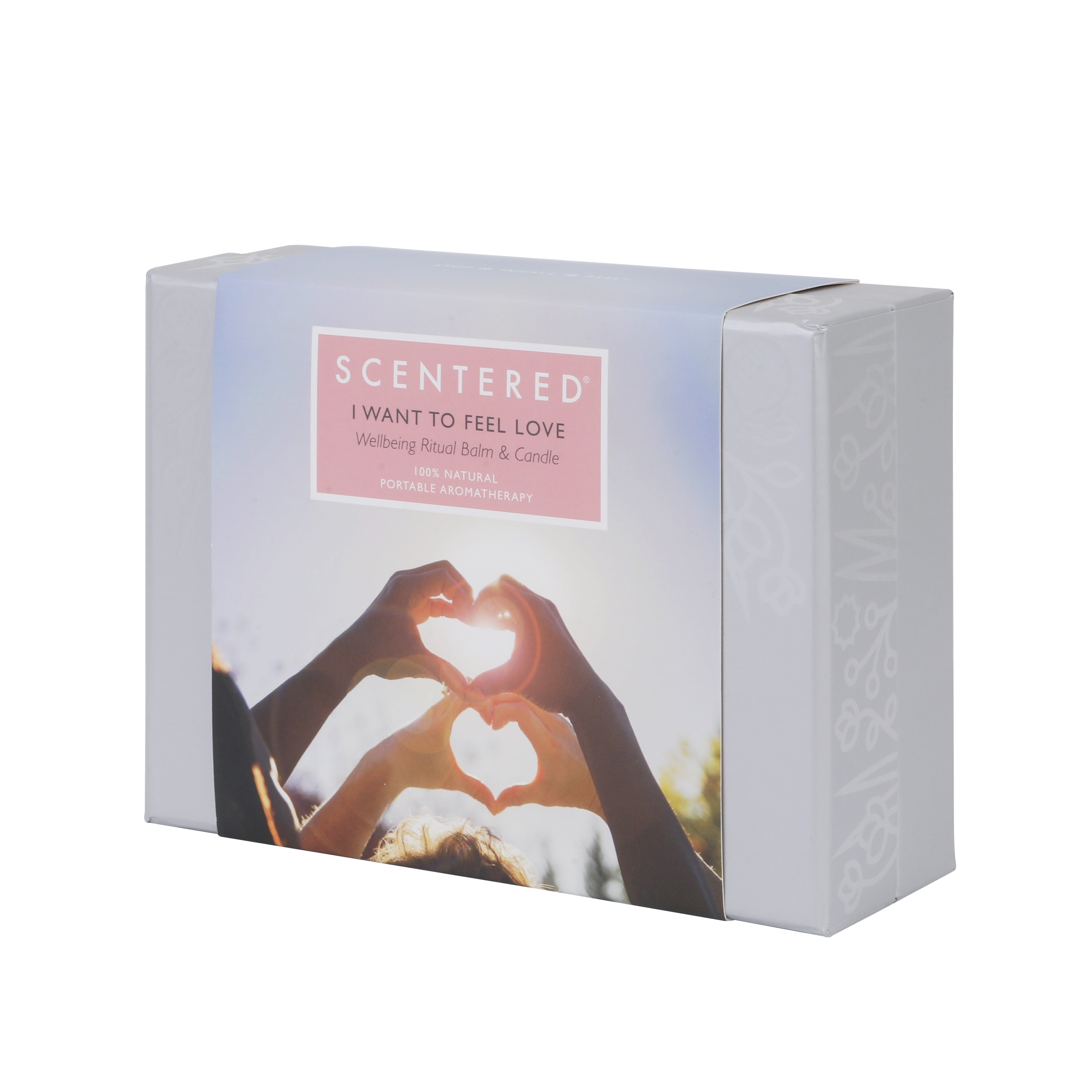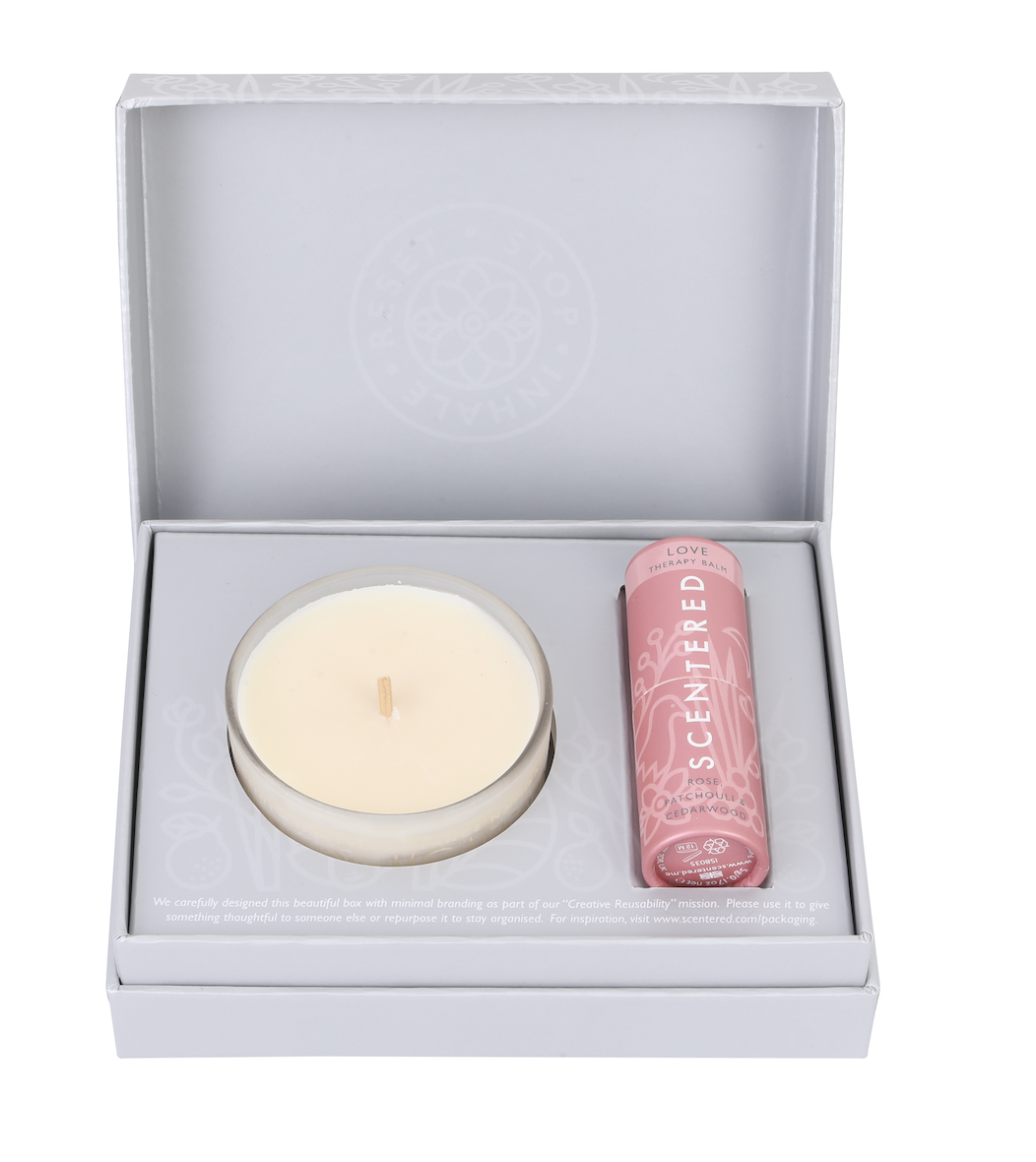### I\$ W\$a\$n\$t\$ T\$o\$ F\$e\$e\$l\$ L\$

£29.50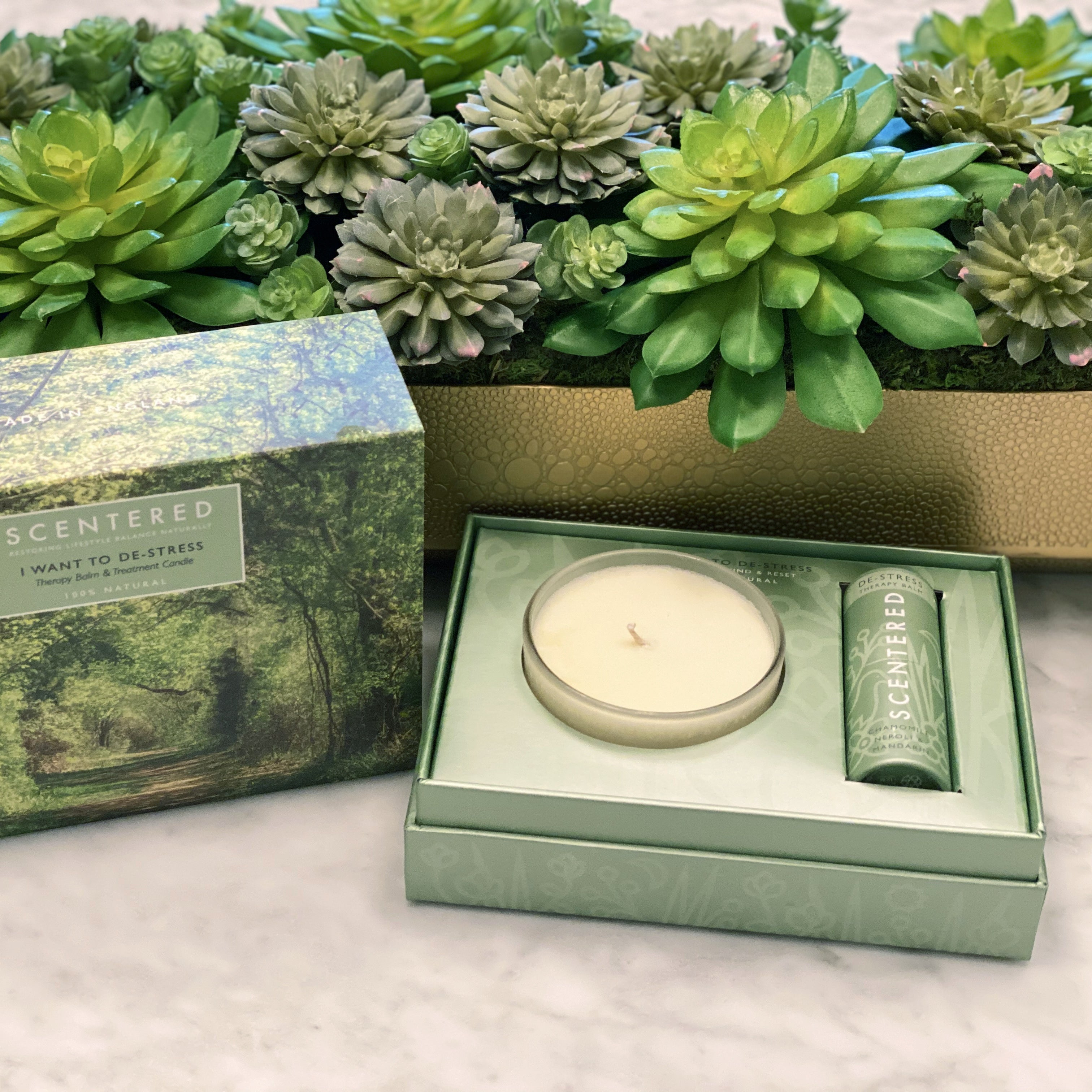### I\$ W\$a\$n\$t\$ T\$o\$ D\$E\$-S\$T\$R\$

£29.50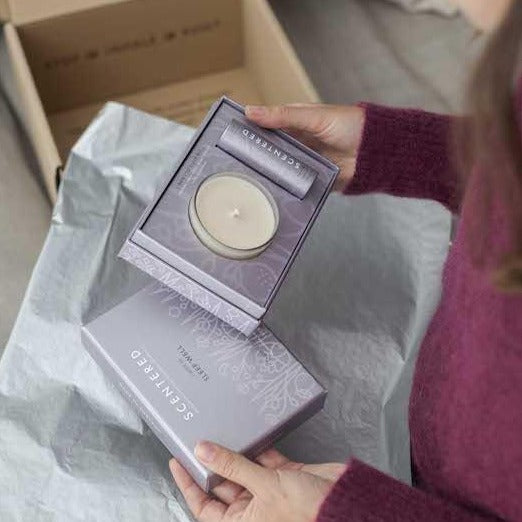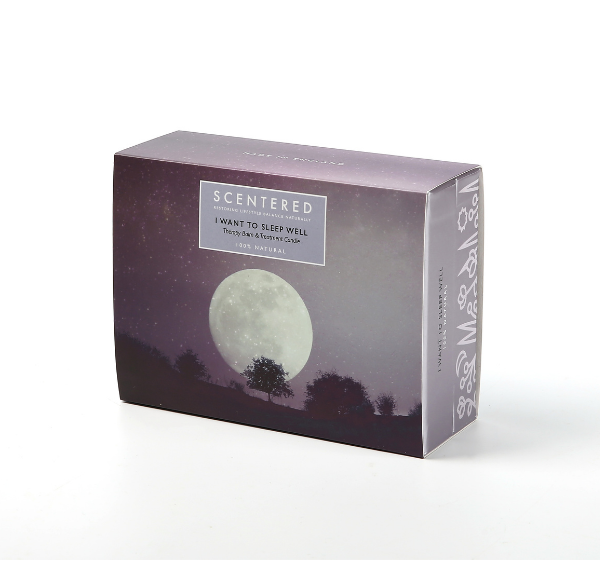### I\$ W\$a\$n\$t\$ T\$o\$ S\$L\$E\$E\$P\$ W

£29.50### I\$ W\$a\$n\$t\$ T\$o\$ E\$S\$C\$A\$

£29.50### H\$o\$m\$e\$ S\$p\$a\$ D\$e\$ S\$t\$r\$e\$s\$s\$

£29.50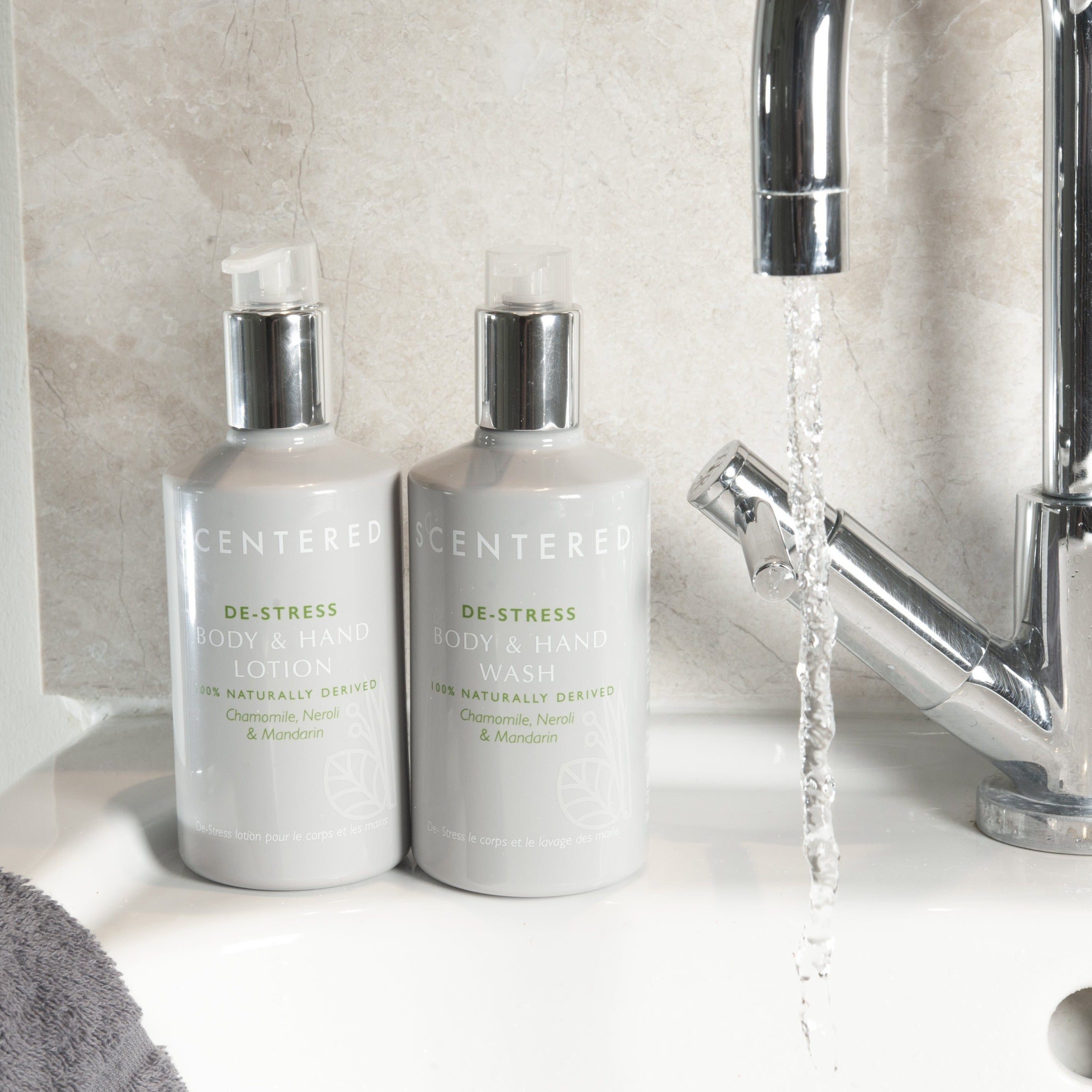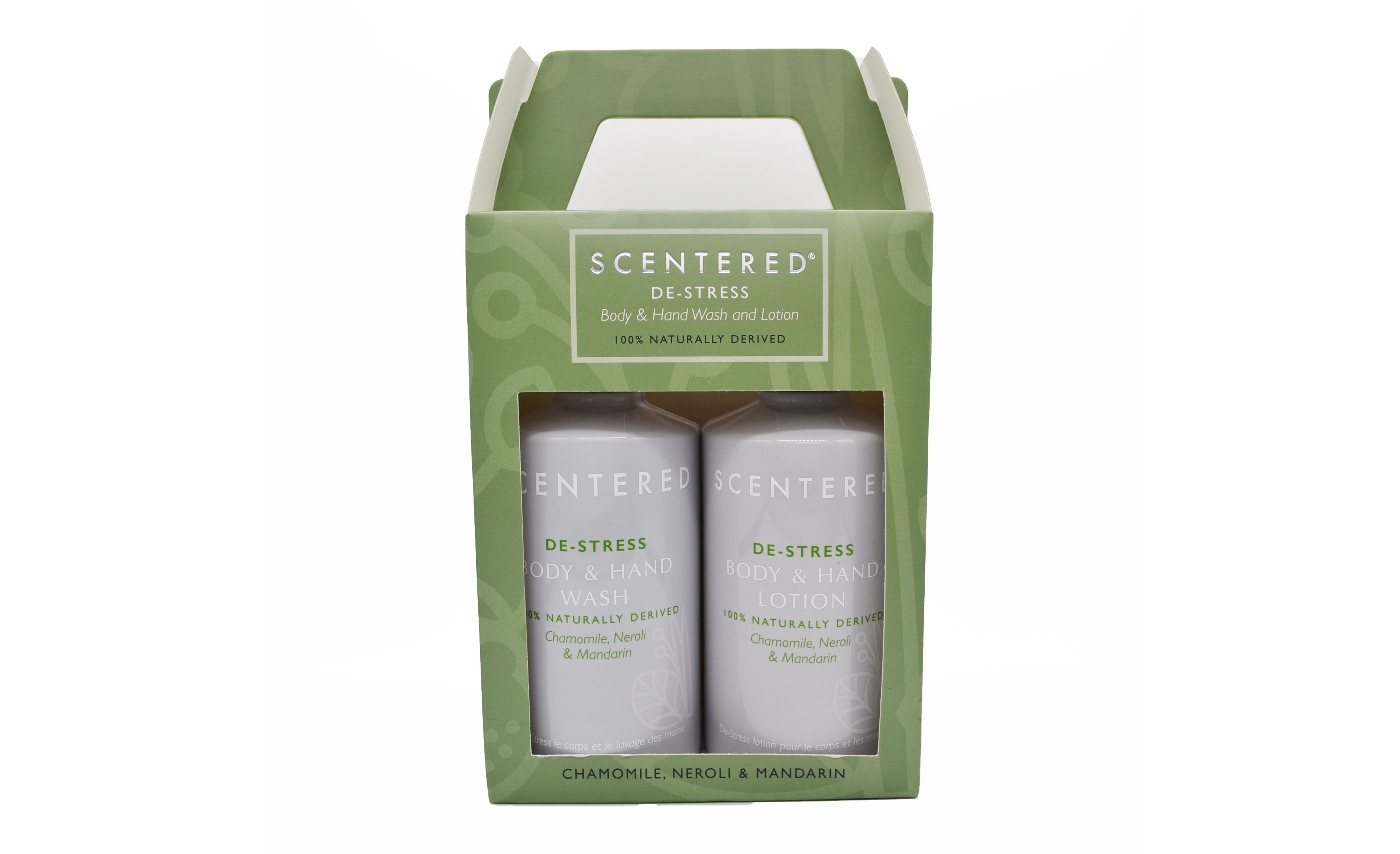### D\$E\$-S\$T\$R\$E\$S\$S\$ W\$a\$s\$h\$

£29.00### D\$E\$-S\$T\$R\$E\$S\$S\$

£17.00### D\$E\$-S\$T\$R\$E\$S\$S\$ C\$o

£17.00### D\$E\$-S\$T\$R\$E\$S\$S\$ B\$o\$d\$y\$ a

£16.00### L\$u\$x\$u\$r\$y\$ W\$e\$l\$l\$b\$e\$i\$n\$g\$ S\$l\$

£29.00### Sleep Well & De Stress Aromatherapy Balm Duo

£27.00### G\$i\$f\$t\$

De £10.00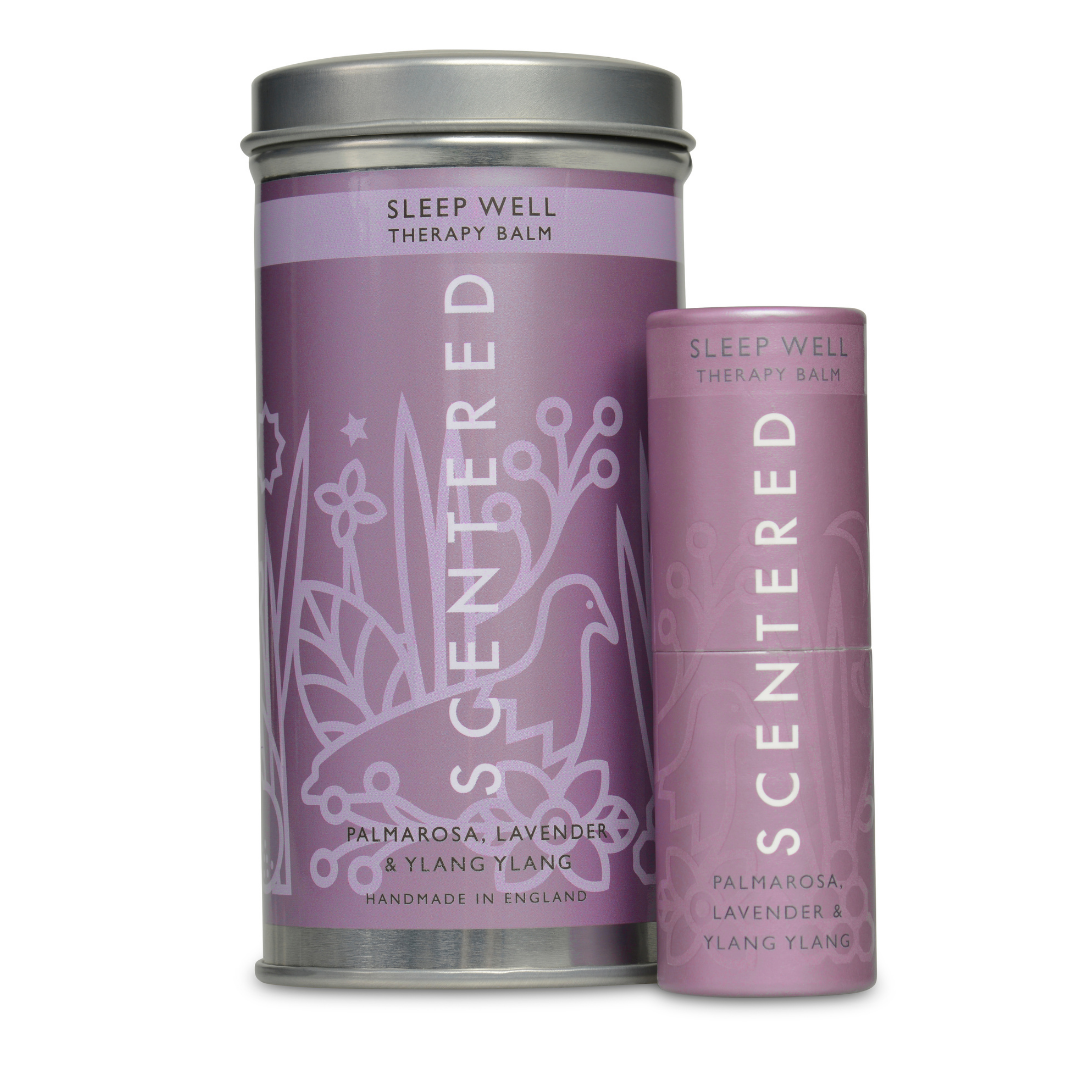### S\$L\$E\$E\$P\$ W\$E\$L\$L\$ W\$e\$l\$l\$b\$e\$i\$n\$g\$ R\$i\$t\$u\$a\$l\$ A\$r\$o\$

£17.90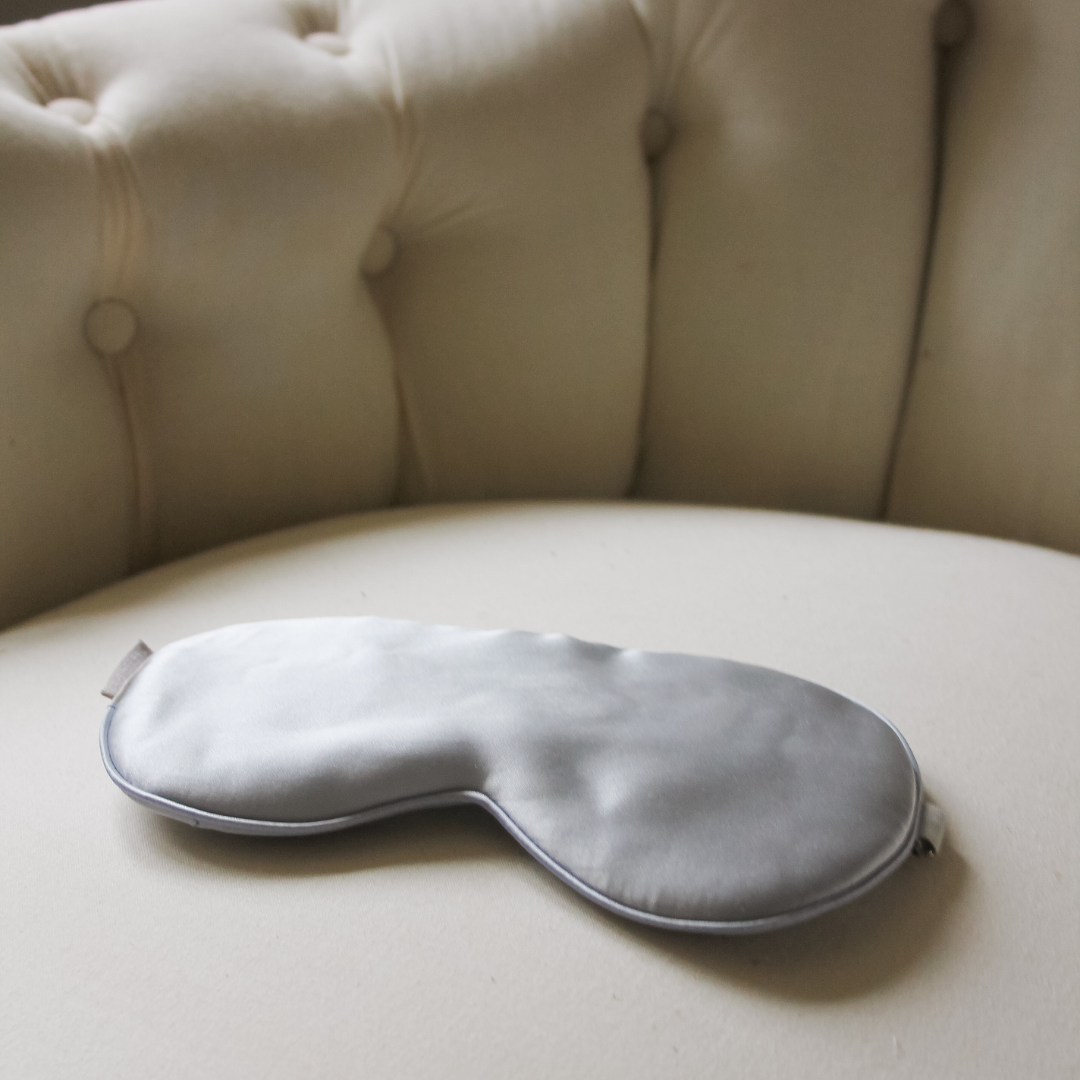### S\$i\$l\$k\$ E\$y\$

£19.99£16.95